International
Tables for
Crystallography
Volume B
Reciprocal space
Edited by U. Shmueli

International Tables for Crystallography (2010). Vol. B, ch. 1.3, pp. 27-28   | 1 | 2 |

Section 1.3.2.2.6. Topology in function spaces

G. Bricognea

aGlobal Phasing Ltd, Sheraton House, Suites 14–16, Castle Park, Cambridge CB3 0AX, England, and LURE, Bâtiment 209D, Université Paris-Sud, 91405 Orsay, France

1.3.2.2.6. Topology in function spaces

| top | pdf |

Geometric intuition, which often makes obvious' the topological properties of the real line and of ordinary space, cannot be relied upon in the study of function spaces: the latter are infinite-dimensional, and several inequivalent notions of convergence may exist. A careful analysis of topological concepts and of their interrelationship is thus a necessary prerequisite to the study of these spaces. The reader may consult Dieudonné (1969, 1970), Friedman (1970), Trèves (1967)and Yosida (1965)for detailed expositions.

1.3.2.2.6.1. General topology

| top | pdf |

Most topological notions are first encountered in the setting of metric spaces. A metric space E is a set equipped with a distance function d fromto the non-negative reals which satisfies: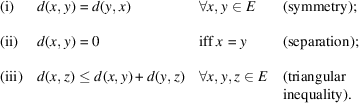By means of d, the following notions can be defined: open balls, neighbourhoods; open and closed sets, interior and closure; convergence of sequences, continuity of mappings; Cauchy sequences and completeness; compactness; connectedness. They suffice for the investigation of a great number of questions in analysis and geometry (see e.g. Dieudonné, 1969).

Many of these notions turn out to depend only on the properties of the collectionof open subsets of E: two distance functions leading to the samelead to identical topological properties. An axiomatic reformulation of topological notions is thus possible: a topology in E is a collectionof subsets of E which satisfy suitable axioms and are deemed open irrespective of the way they are obtained. From the practical standpoint, however, a topology which can be obtained from a distance function (called a metrizable topology) has the very useful property that the notions of closure, limit and continuity may be defined by means of sequences. For nonmetrizable topologies, these notions are much more difficult to handle, requiring the use of filters' instead of sequences.

In some spaces E, a topology may be most naturally defined by a family of pseudo-distances, where eachsatisfies (i) and (iii) but not (ii). Such spaces are called uniformizable. If for every pairthere existssuch that, then the separation property can be recovered. If furthermore a countable subfamily of thesuffices to define the topology of E, the latter can be shown to be metrizable, so that limiting processes in E may be studied by means of sequences.

1.3.2.2.6.2. Topological vector spaces

| top | pdf |

The function spaces E of interest in Fourier analysis have an underlying vector space structure over the fieldof complex numbers. A topology on E is said to be compatible with a vector space structure on E if vector addition [i.e. the map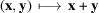] and scalar multiplication [i.e. the map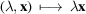] are both continuous; E is then called a topological vector space. Such a topology may be defined by specifying a `fundamental system S of neighbourhoods of', which can then be translated by vector addition to construct neighbourhoods of other points.

A norm ν on a vector space E is a non-negative real-valued function onsuch that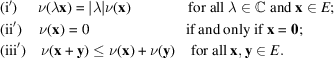Subsets of E defined by conditions of the formwith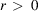form a fundamental system of neighbourhoods of 0. The corresponding topology makes E a normed space. This topology is metrizable, since it is equivalent to that derived from the translation-invariant distance. Normed spaces which are complete, i.e. in which all Cauchy sequences converge, are called Banach spaces; they constitute the natural setting for the study of differential calculus.

A semi-norm σ on a vector space E is a positive real-valued function onwhich satisfies (i′) and (iii′) but not (ii′). Given a set Σ of semi-norms on E such that any pair (x, y) inis separated by at least one, let B be the set of those subsetsof E defined by a condition of the formwithand; and let S be the set of finite intersections of elements of B. Then there exists a unique topology on E for which S is a fundamental system of neighbourhoods of 0. This topology is uniformizable since it is equivalent to that derived from the family of translation-invariant pseudo-distances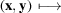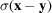. It is metrizable if and only if it can be constructed by the above procedure with Σ a countable set of semi-norms. If furthermore E is complete, E is called a Fréchet space.

If E is a topological vector space over, its dualis the set of all linear mappings from E to(which are also called linear forms, or linear functionals, over E). The subspace ofconsisting of all linear forms which are continuous for the topology of E is called the topological dual of E and is denoted E′. If the topology on E is metrizable, then the continuity of a linear formatcan be ascertained by means of sequences, i.e. by checking that the sequence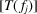of complex numbers converges to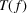inwhenever the sequence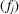converges to f in E.

References

Dieudonné, J. (1969). Foundations of Modern Analysis. New York, London: Academic Press.
Dieudonné, J. (1970). Treatise on Analysis, Vol. II. New York, London: Academic Press.
Friedman, A. (1970). Foundations of Modern Analysis. New York: Holt, Rinehart & Winston. [Reprinted by Dover, New York, 1982.]
Trèves, F. (1967). Topological Vector Spaces, Distributions, and Kernels. New York, London: Academic Press.
Yosida, K. (1965). Functional Analysis. Berlin: Springer-Verlag.